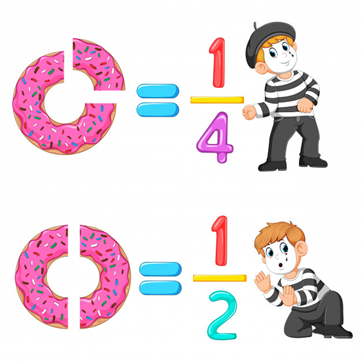Math & Beyond
Real World Applications of Fractions
153.7k views

 1 Introduction 2 What are fractions? 3 Fractions in Mathematics 4 Fractions in the real world 5 Summary 6 FAQs

21 October 2020

## Introduction

Why should I learn about fractions?

There are several ways we use fractions unknowingly while performing our day to day activities. It is fair to say that the world would not be without fractions as we see it today. This blog will shed light on fractions and how they are applied in different mathematics branches and how they impact our everyday life.## What are Fractions?

A fraction is a part of a whole. In other words, a fraction is a number that represents a whole number.

For example, a cake is divided into 8 equal slices. If a single slice is eaten, how can we represent the remaining portion of the cake?

The remaining portion of the cake can be expressed numerically as $$\frac{7}{8}$$.Here, 7 is the numerator, and 8 is the denominator.

## Fractions in Mathematics

Fractions are an elementary topic in mathematics that act as a building block in many other mathematics topics.

Some of the ways fractions are used in mathematics are:

• We can determine the part of any number using fractions.

Example

$\frac{3}{4}\; \text{part of }24 =\frac{3}{4} × 24 = 18$

$\text{Half of }90 = \frac{1}{2}× 90 = 45$

• Fractions are used in determining the decimal and percentages of numbers

Example

\begin{align}\frac{3}{4}× 100 &= 75\% \\\frac{3}{4}&= 0.75\\[6pt]\frac{3}{8}&= 0.375 = 37.5\%\end{align}

• Fractions are used in calculating probability. They are used to attribute a numerical value to the likelihood of the occurrence of an event.

Example

What is the likelihood of the number 3 showing up on rolling an unbiased dice?

The probability that 3 will show up is $$\frac{1}{6}$$

• We also use fractions in algebra when we solve algebraic equations.
• Fractions are used in ratios.
• These concepts along with others are widely used in mathematics and other sciences to perform calculations.

## Fractions in the real world

Fractions surround our everyday activities. Here are some examples of fractions in real life:

• Eating at a restaurant: Think about a time you go to a restaurant with friends and the waitress brings a single bill. To divide the total amongst the friends, you use fractions.

• Shopping: Think about the time you went shopping for a new school bag. There was half off on everything due to a sale, so you calculate the new price using fractions.

• Following a recipe: Recipes sometimes suggest using $$\frac{1}{2}$$ a teaspoon of sugar, $$\frac{3}{4}$$ tablespoon of salt. Some recipes also have quantities to serve 2. If we are making the same dish to serve 3 people, fractions are used to adjust the ingredients accordingly.

• Sports: Fractions are frequently used to analyze the performance of a particular player and team.

• Fitness: We use fractions to understand our body mass index (BMI) to determine whether we are in a healthy range of body mass or not.

• Drinks: To make drinks like mocktails, different fractions of liquids are mixed in the right amounts to get the best outcome.

• Pizza: Dividing the pizza slices equally amongst everyone requires fractions.

• Photography and videography: The shutter speed of a camera is calculated using fractions.

• Tests and exams: Scores of tests and exams are generally expressed as fractions, like $$\frac{18}{20}$$.

• Medical Prescriptions: When someone is sick, the doctor prescribes different dosages for people of various sizes. A full-grown adult may consume 500mg whereas a child may consume half of that.

• Progress or decline: Progress or decline of any project can be measured. If the sales of a particular product are down by 25% or by $$\frac{1}{4}$$th.

• Time: Half n hour is a common way of expressing 30 minutes.## Summary

The applications of fractions in everyday life are endless; they are of great importance and profoundly impact everything around us. This blog talks about some of the different things involving fractions. Think about some other ways in which fractions are involved in your life.

## What is a fraction?

A fraction is a part of a whole. In other words, a fraction is a number that represents a whole number.

Where are fractions used in mathematics?

Fractions are used in:

• Determining the part of a number

• Calculating decimals and percentages

• Ratio and proportion

• Probability

• Algebraic equations

## What are some examples of fractions in real life?

Here are some applications of fractions in real life:

• Splitting a bill while eating at a restaurant

• Calculating the discounted price of an object on sale.

• Following a recipe

• Fractions are frequently used to analyze the performance of a particular player and team.

• We use fractions to understand our body mass index (BMI) to determine whether we are in a healthy range of body mass or not.

• Different fractions of liquids are mixed in the right amounts to make mocktails.

• Dividing pizza slices equally amongst everyone requires fractions.

• The shutter speed of a camera is calculated using fractions.

• Scores of tests and exams are generally expressed as fractions, like $$\frac{18}{20}$$.

• The doctor prescribes different dosages, for people of different sizes based on fractions.

• Progress or decline of any project can be measured. If the sales of a particular product are down by 25% or by $$\frac{1}{4}$$ th.

• In time, Half n hour is a common way of expressing 30 minutes.

## How to multiply fractions?

To multiply fractions, we multiply the two numerators and the two denominators with one another.

Example

$\frac{2}{3}\times\frac{4}{5}=\frac{2\times3}{4\times 5}=\frac{8}{15}$

Related Articles